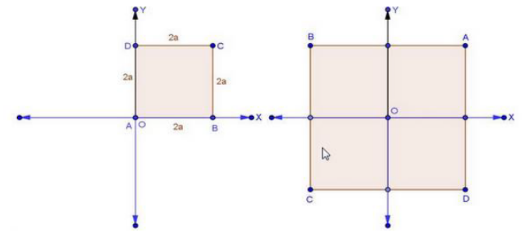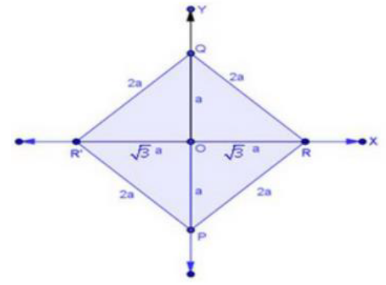×#### Thank you for registering.

One of our academic counsellors will contact you within 1 working day.

Click to Chat

1800-1023-196

+91-120-4616500

CART 0

• 0

MY CART (5)

Use Coupon: CART20 and get 20% off on all online Study Material

ITEM
DETAILS
MRP
DISCOUNT
FINAL PRICE
Total Price: Rs.

There are no items in this cart.
Continue Shopping• Complete JEE Main/Advanced Course and Test Series
• OFFERED PRICE: Rs. 15,900
• View Details

```Chapter 14: Coordinate Geometry Exercise – 14.1

Question: 1

On which axis do the following points lie?

(i) P (5,0)

(ii) Q (0 — 2)

(iii) R (-4, 0)

(iv) S (0, 5)

Solution:

(i) P (5, 0) lies on x – axis

(ii) Q (0, -2) lies on y – axis

(iii) R (-4, 0) lies on x – axis

(iv) S (0, 5) lies on y – axis

Question: 2

Let ABCD be a square of side 2a. Find the coordinates of the vertices of this square when

(i) A coincides with the origin and AB and AB and coordinate axes are parallel to the sides AB and AD respectively.

(ii) The center of the square is at the origin and coordinate axes are parallel to the sides AB and AD respectively.Solution:

(i) Coordinate of the vertices of the square of side 2a are:

A (0, 0), B (2a, 0), C (2a, 2a) and D (0, 2a)

(ii) Coordinate of the vertices of the square of side 2a are:

A (a, a), B (- a, a), C (- a, a) and (a, – a)

Question: 3

The base PQ of two equilateral triangles PQR and PQR' with side 2a lies along y-axis such that the mid-point of PQ is at the origin. Find the coordinates of the vertices R and R' of the triangles.Solution:

We have two equilateral triangle PQR and PQR' with side 2a.

O is the mid-point of PQ.

In ∆Q0R. ∠QOR = 90°

Hence, by Pythagoras theorem

OR2 + OQ2 = QR2

OR2 = (2a)2 – (a)2

OR2 = 3a2

OR = √(3) a

Coordinates of vertex R is (√3a, 0) and coordinate of vertex R' is (- √3a, 0)
```### Course Features

• 728 Video Lectures
• Revision Notes
• Previous Year Papers
• Mind Map
• Study Planner
• NCERT Solutions
• Discussion Forum
• Test paper with Video Solution